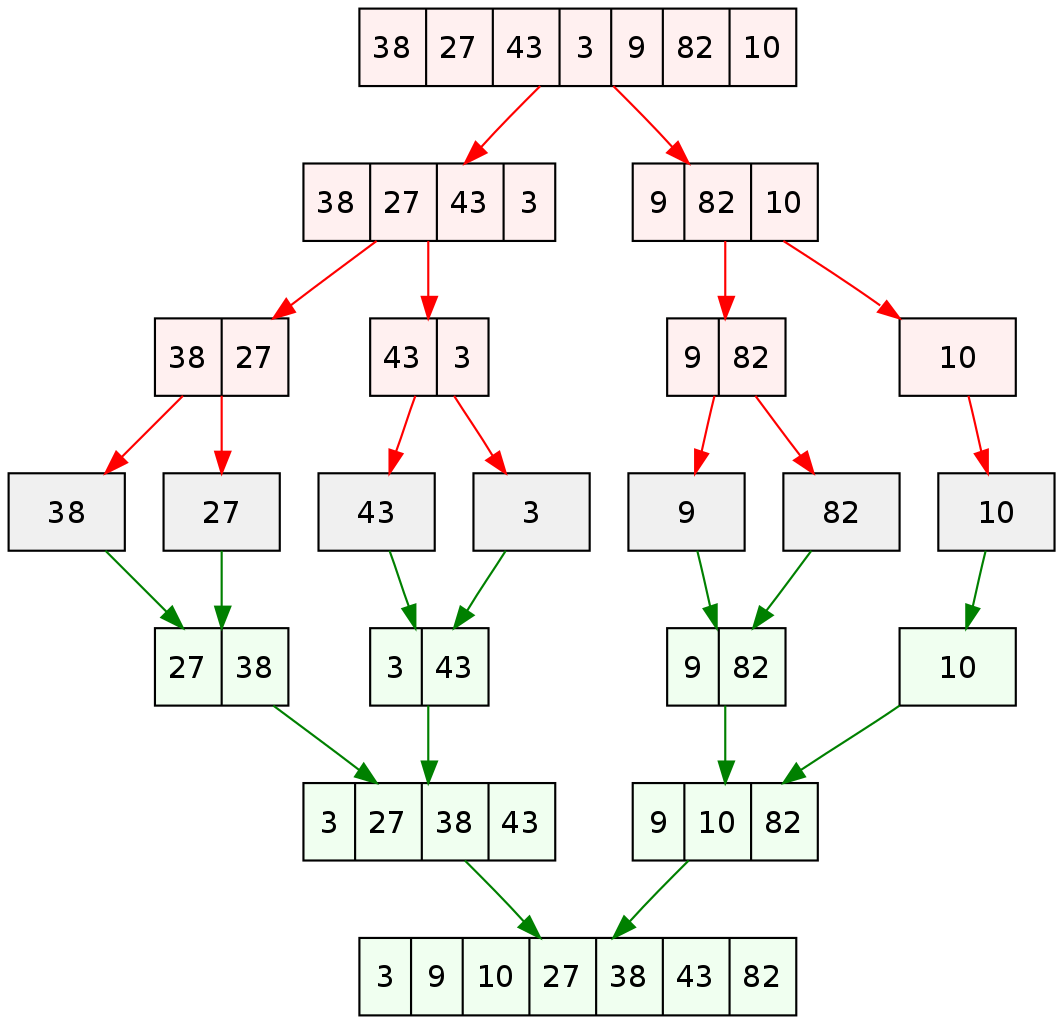# Sorting Algorithm - Merge sort Implementation

The 4th sort algorithm I am going to show today is Merge Sort.

## Merge sort

Merge Sort uses the Divide and Conquer algorithm because it involves splitting the array into sub-arrays.

There are two phases in Merge Sort: Splitting and Merging. We do the sorting during the merging phase.

The splitting phase is the preparation phase to make sorting faster during the merging phase.## How does it work?

### Splitting Phase

Start with an unsorted array, then we divide the array into 2 sub-arrays, and each sub-array we continue to divide into smaller arrays and so on. Until all the arrays only have one element (considered sorted)

### Merging Phase

We take a bunch of one element arrays and then merging them into 2-element arrays, then 4–elements array and so on.

Start to merge from bottom to up. Merge the sibling arrays (left | right air). Each merge, making sure the resulting arrays are sorted. Until all arrays are merged into one, it means the array is sorted.

## Implementation

### Ascending

• Initial a temporary array large enough to hold all elements in the arrays. E.g: first round, the temp array length equals 2

• To merge sibling arrays left and right, we compare the value index from each array

• i: first index of left array (not start from 0, the index of element getting from the orignial array)
• j: first index of right array (not start from 0, the index of element getting from the original array)
• if left[i] <= right[j] then copy left[i] into temp and increase i (i++)
• if left[i] > right[j] then copy right[j] into temp and increase j (j++)
• We compare to get the smallest value between left and right and copying it into temp array. Repeat until all elements in 2 arrays have been processed. It means the temp array now contains merged values in sorted order.

• Then we copy the temp array back to original input array at the correct positions. Now the original array has changed - it contains few elements in sorted order.

• We keep do it this until the original array contains all elements in sorted order.